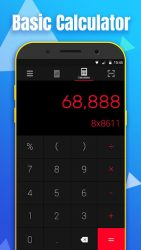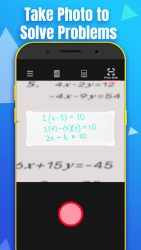# Download Math Calculator-Solve Math Problems by Camera 1.7.3 for Android - APK DownloadMath Calculator-Solve Math Problems by Camera ScreenShot1Math Calculator-Solve Math Problems by Camera ScreenShot2

Unlock Math Calculator to do maths solutions by taking photo! Math Calculator is a useful and easy-to-use calculator pro with basic calculator free and scientific calculator. This stylish calculator is also an equation solver and a great maths homework solver which can solve maths problems more efficiently by CAMERA . This calculator app is developed by a experienced team which has already created a calculator app with 1,000,000+ install volume. We still devote to developing the best calculator app. DOWNLOAD it right now and try it for FREE! Whats inside Basic Calculator: Basic mathematics free functions with percentage. Maths Camera: Good maths homework helper which solves maths problems by taking photo. Solve Multiple Problems: Solve multi-line math problems at one time. Correct wrong answers: Check your answers and correct the wrong ones. Scientific Calculator: Based on casio 570. Calculation history : Never lose your calculations Equation calculator: Solve your maths homework efficiently. Great design: Nice user interface based on gesture. Floating Window: eep calculating above other apps. Vibrate and Sound: Fashion functions that simulates a real calculator.

#### Additional information:

Application Name:
Math Calculator-Solve Math Problems by Camera
Version:
1.7.3
Developed By:
Super T Group
File Size:
7.43 MB
Category:
Business
Date:
01/12/2019

# Reviewer overview

## Math Calculator-Solve Math Problems by Camera Review

Please review Math Calculator-Solve Math Problems by Camera application and submit your comments below. We will collect all comments in an effort to determine whether the Math Calculator-Solve Math Problems by Camera app is reliable, perform as expected and deliver the promised features and functionalities.

5

Popularity 5/10

Downloads - 37

Score - 5